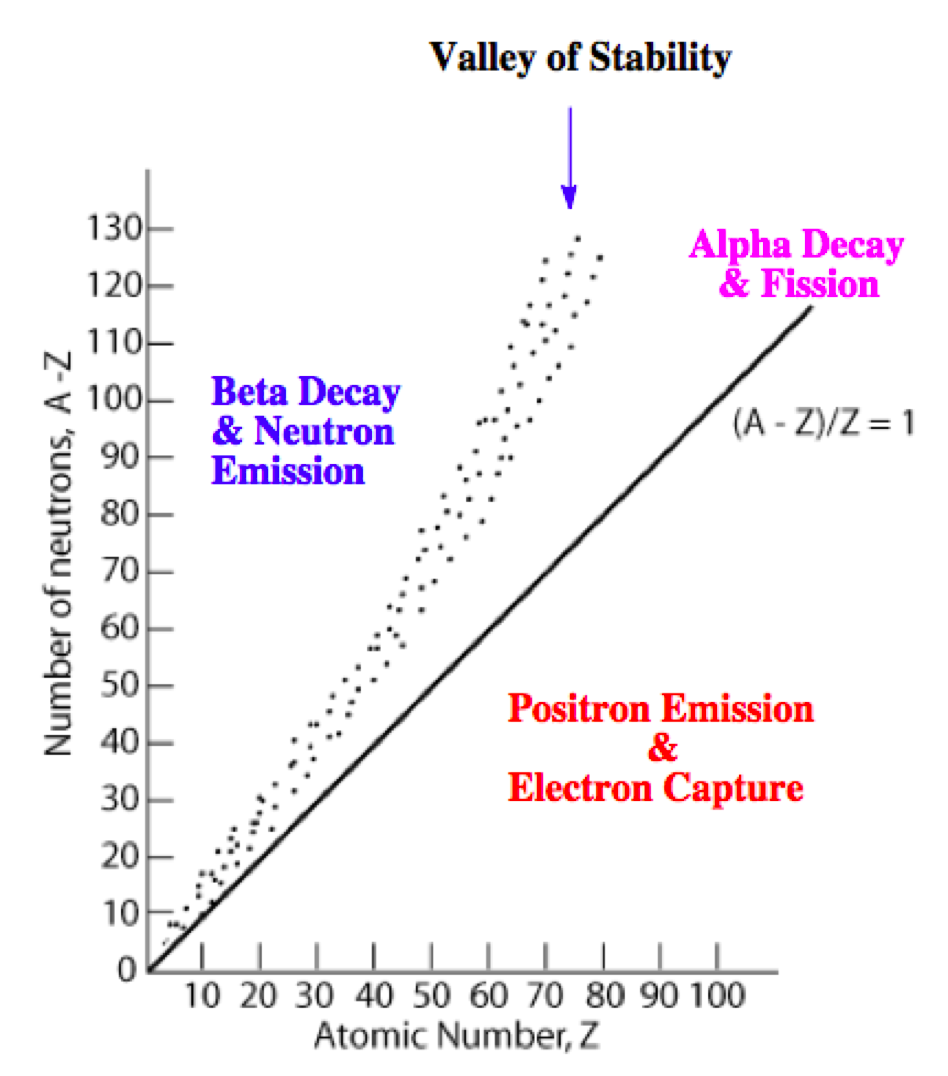# Problem: Predict whether each nuclide is more likely to decay via beta decay or positron emission.(a) Pb-192(b) Pb-212(c) Xe-114

###### FREE Expert Solution

We’re being asked to predict the most likely mode of decay for each of the following radioactive isotopes

Recall that the stability of an isotope depends on the ratio of neutrons to protons (N/Z). This means for stable isotopes with:

• Z ≤ 20; the N/Z ratio should be equal to 1.0

• 20 < Z ≤ 40; the N/Z ratio should be equal to 1.25

• 40 < Z ≤ 80; the N/Z ratio should be equal to 1.50

• Z < 83; the stable nuclide does not exist

The N/Z values for stable isotopes form the valley or band of stability:• above the valley of stability: there too many neutrons in the isotope
• Mode of decay: Either beta decay (0–1β) or neutron emission (10n)
97% (342 ratings)###### Problem Details

Predict whether each nuclide is more likely to decay via beta decay or positron emission.
(a) Pb-192
(b) Pb-212
(c) Xe-114